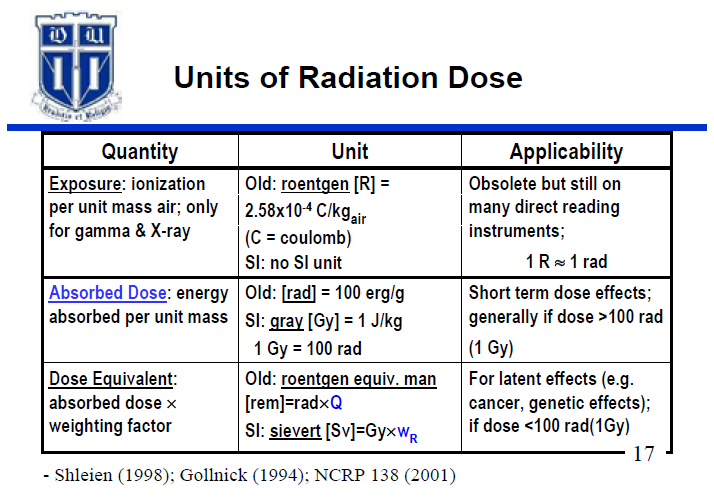## Pages

### Units of Radiation

The roentgen was one of the earliest (1928) units used to measure exposure and measures
the amount of ionization produced in air by X-ray or gamma rays. By definition,
1 R = 2.58 × 10^-4 coulombs/kilogram at standard temperature and pressure. It is important to note that the unit of exposure is defined only for X or gamma rays and only for exposure in air. Thus, the
roentgen is a measure of the ability of photons to ionize air. One roentgen (R) is a rather large
exposure, so frequently the milliroentgen (mR) unit is used (1000 mR = 1 R). Many Geiger
counters and most ion chambers are calibrated to read out in terms of exposure rate, e.g.,
milli roentgen per hour (mR/hr). There is no international system (SI) unit for exposure.
Radiation deposits energy when absorbed by matter. This energy deposition leads to the
biological effect of ionizing radiation. By definition, the absorbed dose is the energy deposited
per unit mass. The unit of radiation absorbed dose is called the rad. One rad represents the
deposition of 100 ergs per gram of material. Unfortunately, the rad is difficult to measure and
must often be calculated from other measurements. However, for radiation protection purposes,
1 R = 1 rad, for X-rays or gamma rays. But, for beta radiations, 1 R equals some constant times
1 rad, where the constant is dependent upon the beta energy. For example: 1 R ≅ 2.6 rad for
P-32. Therefore, beta measurements with survey meters which read in R/hr or mR/hr must be
interpreted with care. The international system (SI) unit for absorbed dose is the gray (Gy) –
one Gy = 100 rad.
The ultimate aim of a dose measurement system, from a radiation safety viewpoint, is to arrive
at a quantity appropriate for predicting biological response independent of the source of the
radiation. This goal is only partially achieved with the rad. For example, the biological effect is
much greater for alpha radiation than for beta or gamma radiation for a given absorbed dose (in
rad) to the biological system. This difference in the biological effectiveness of the radiation has
been attributed primarily to the fact that alpha radiation releases more energy and ionizes more
particles per unit path length traversed than beta or gamma although the path length is shorter
than that for beta or gamma radiations. In order to account for the different biological effects of
different radiation types, a quality factor (QF) has been introduced which is used to convert the
absorbed dose to a dose equivalent. Quality factors are shown in slide 18. The unit of dose
equivalent is the rem (Roentgen - Equivalent - Mammal.).
Most biomedical research laboratory situations involve only beta, gamma, or X-ray radiations.
For these radiations, the QF is equal to 1 so the dose equivalent (in rem) is equal to the
absorbed dose (in rad). The dose to tissue in air from exposure to one Roentgen is about 0.95
rad. Therefore, for X-ray and gamma radiation exposure, the following expression
approximates the dose equivalent:

1 R ≈1 rad = 1 rem [Low LET radiation]

One rem is a large unit; so, we usually work in terms of millirem (mrem),
where 1000 mrem = 1 rem. The SI unit for rem is the sievert (Sv) – one Sv = 100 rem